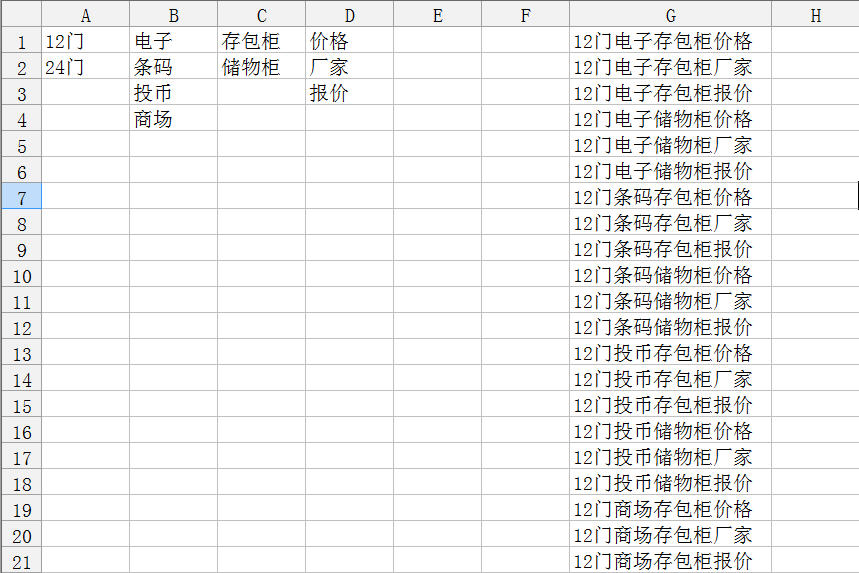Home >  > 利用Excel生成关键词列表

利用Excel生成关键词列表

0

12门
24门

Excel宏代码如下：

Sub a()

Dim a, b, c, d As Integer

a = Sheets(1).[a65536].End(xlUp).Row

b = Sheets(1).[b65536].End(xlUp).Row

c = Sheets(1).[c65536].End(xlUp).Row

d = Sheets(1).[d65536].End(xlUp).Row

For i = 1 To a

For p = 1 To b

For q = 1 To c

For t = 1 To d

x = x + 1

Cells(x, 7).Value = Cells(i, 1).Value & Cells(p, 2).Value & Cells(q, 3).Value & Cells(t, 4).Value

Next

Next

Next

Next

End Sub